# Bicycle dynamics

The bicycle is an interesting dynamical system with the feature that one of its key properties is due to a feedback mechanism that is created by the design of the front fork. A detailed model of a bicycle is complex because the system has many degrees of freedom and the geometry is complicated. However, a great deal of insight can be obtained from simple models.

## Modeling

The diagrams at the top of the page show the key parameters for the bicycle. The equations of motion can be derived by keeping track of the balance of forces acting on the system: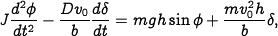where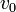is the forward velocity of the bicycle.

The Whipple model of the bicycle can be computed by using the rigid-body dynamics of the front fork and frame. The linearized model (around the upright position, with steering wheel straight) is given by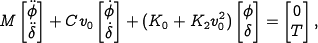where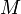,,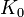andare 2x2 matrices that depend on the geometry and mass distribution of the bicycle. A representative set of parameter values for these matrices are available in the file bicycle_whipple.m.

Exercise: Combine the bicycle model given above and the model for stering kineamatics in Chapter 2 - System Modeling to obtain a model that describes the path of the center of mass of the bicycle.